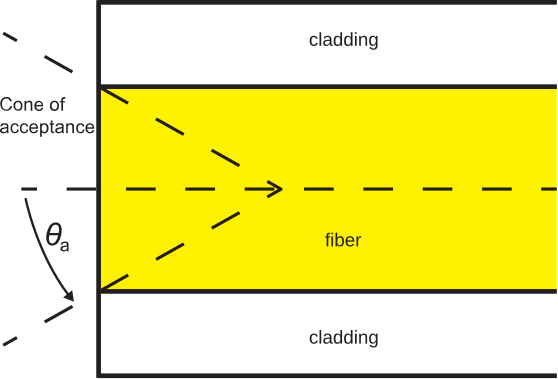# 8.2: Acceptance Angle

$$\newcommand{\vecs}{\overset { \rightharpoonup} {\mathbf{#1}} }$$ $$\newcommand{\vecd}{\overset{-\!-\!\rightharpoonup}{\vphantom{a}\smash {#1}}}$$$$\newcommand{\id}{\mathrm{id}}$$ $$\newcommand{\Span}{\mathrm{span}}$$ $$\newcommand{\kernel}{\mathrm{null}\,}$$ $$\newcommand{\range}{\mathrm{range}\,}$$ $$\newcommand{\RealPart}{\mathrm{Re}}$$ $$\newcommand{\ImaginaryPart}{\mathrm{Im}}$$ $$\newcommand{\Argument}{\mathrm{Arg}}$$ $$\newcommand{\norm}{\| #1 \|}$$ $$\newcommand{\inner}{\langle #1, #2 \rangle}$$ $$\newcommand{\Span}{\mathrm{span}}$$ $$\newcommand{\id}{\mathrm{id}}$$ $$\newcommand{\Span}{\mathrm{span}}$$ $$\newcommand{\kernel}{\mathrm{null}\,}$$ $$\newcommand{\range}{\mathrm{range}\,}$$ $$\newcommand{\RealPart}{\mathrm{Re}}$$ $$\newcommand{\ImaginaryPart}{\mathrm{Im}}$$ $$\newcommand{\Argument}{\mathrm{Arg}}$$ $$\newcommand{\norm}{\| #1 \|}$$ $$\newcommand{\inner}{\langle #1, #2 \rangle}$$ $$\newcommand{\Span}{\mathrm{span}}$$$$\newcommand{\AA}{\unicode[.8,0]{x212B}}$$

In this section, we consider the problem of injecting light into a fiber optic cable. The problem is illustrated in Figure $$\PageIndex{1}$$.Figure $$\PageIndex{1}$$: Injecting light into a fiber optic cable. ( CC BY-SA 4.0; S. Lally)

In this figure, we see light incident from a medium having index of refraction $$n_0$$, with angle of incidence $$\theta^i$$. The light is transmitted with angle of transmission $$\theta_2$$ into the fiber, and is subsequently incident on the surface of the cladding with angle of incidence $$\theta_3$$. For light to propagate without loss within the cable, it is required that

$\sin\theta_3 \ge \frac{n_c}{n_f} \label{m0192_eCAnm}$

since this criterion must be met in order for total internal reflection to occur.

Now consider the constraint that Equation \ref{m0192_eCAnm} imposes on $$\theta^i$$. First, we note that $$\theta_3$$ is related to $$\theta_2$$ as follows:

$\theta_3 = \frac{\pi}{2} - \theta_2 \nonumber$

therefore

\begin{align} \sin\theta_3 &= \sin\left(\frac{\pi}{2} - \theta_2\right) \\ &= \cos\theta_2 \end{align}

so

$\cos\theta_2 \ge \frac{n_c}{n_f} \nonumber$

Squaring both sides, we find:

$\cos^2\theta_2 \ge \frac{n_c^2}{n_f^2} \nonumber$

Now invoking a trigonometric identity:

$1-\sin^2\theta_2 \ge \frac{n_c^2}{n_f^2} \nonumber$

so:

$\sin^2\theta_2 \le 1-\frac{n_c^2}{n_f^2} \label{m0192_e1}$

Now we relate the $$\theta_2$$ to $$\theta^i$$ using Snell’s law:

$\sin\theta_2 = \frac{n_0}{n_f}\sin\theta^i \nonumber$

so Equation \ref{m0192_e1} may be written:

$\frac{n_0^2}{n_f^2}\sin^2\theta^i \le 1-\frac{n_c^2}{n_f^2} \nonumber$

Now solving for $$\sin\theta^i$$, we obtain:

$\sin\theta^i \le \frac{1}{n_0}\sqrt{ n_f^2 - n_c^2 } \nonumber$

This result indicates the range of angles of incidence which result in total internal reflection within the fiber. The maximum value of $$\theta^i$$ which satisfies this condition is known as the acceptance angle $$\theta_a$$, so:

$\theta_a \triangleq \arcsin\left(\frac{1}{n_0}\sqrt{ n_f^2 - n_c^2 }\right) \nonumber$

This leads to the following insight:

In order to effectively launch light in the fiber, it is necessary for the light to arrive from within a cone having half-angle $$\theta_a$$ with respect to the axis of the fiber.

The associated cone of acceptance is illustrated in Figure $$\PageIndex{2}$$.Figure $$\PageIndex{2}$$: Cone of acceptance. ( CC BY-SA 4.0)

It is also common to define the quantity numerical aperture NA as follows:

$\mbox{NA} \triangleq \frac{1}{n_0}\sqrt{ n_f^2 - n_c^2 } \label{m0192_eNA}$

Note that $$n_0$$ is typically very close to $$1$$ (corresponding to incidence from air), so it is common to see NA defined as simply $$\sqrt{ n_f^2 - n_c^2 }$$. This parameter is commonly used in lieu of the acceptance angle in datasheets for fiber optic cable.

##### Example $$\PageIndex{1}$$: Acceptance angle

Typical values of $$n_f$$ and $$n_c$$ for an optical fiber are 1.52 and 1.49, respectively. What are the numerical aperture and the acceptance angle?

###### Solution

Using Equation \ref{m0192_eNA} and presuming $$n_0=1$$, we find NA $$\cong \underline{0.30}$$. Since $$\sin\theta_a =$$ NA, we find $$\theta_a=\underline{17.5^{\circ}}$$. Light must arrive from within $$17.5^{\circ}$$ from the axis of the fiber in order to ensure total internal reflection within the fiber.

This page titled 8.2: Acceptance Angle is shared under a CC BY-SA license and was authored, remixed, and/or curated by Steven W. Ellingson (Virginia Tech Libraries' Open Education Initiative) .